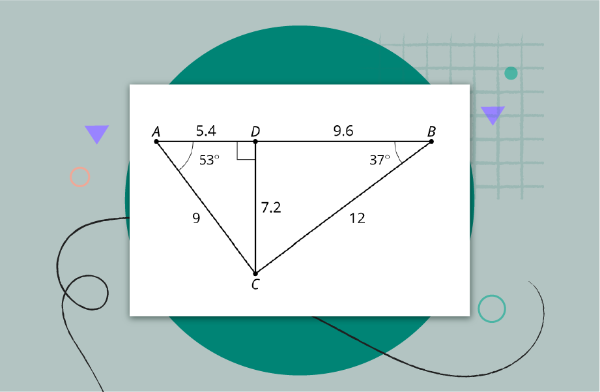Explore

# Geometry: similarity

In this unit, students use dilations and rigid transformations to justify triangle similarity theorems. The unit balances a focus on proof with a focus on using similar triangles to find unknown side lengths and angle measurements.

Year level(s) Year 9
Audience Teacher
Purpose Content knowledge, Teaching resource, Student task
Teaching strategies and pedagogical approaches Explicit teaching
Keywords Transformation, Ratio, Triangle, Angle, Proof, Congruent, Similar, Scale factor, Enlargement

## Curriculum alignment

Curriculum connections Critical and creative thinking, Numeracy
Strand and focus Space
Topics Angles and geometric reasoning
AC: Mathematics (V9.0) content descriptions
AC9M9M03

Solve spatial problems, applying angle properties, scale, similarity, Pythagoras' theorem and trigonometry in right-angled triangles

Numeracy progression Understanding geometric properties (P7)
Proportional thinking (P7)
Understanding units of measurement (P10)